## Introduction¶

Working with these files can be a challenge, especially given their heterogeneous nature. Some preprocessing is required before they are ready for consumption by your CNN.

Fortunately, I participated in the LUNA16 competition as part of a university course on computer aided diagnosis, so I have some experience working with these files. At this moment we top the leaderboard there :)

This tutorial aims to provide a comprehensive overview of useful steps to take before the data hits your ConvNet/other ML method.

What we will cover:

• Converting the pixel values to Hounsfield Units (HU), and what tissue these unit values correspond to
• Resampling to an isomorphic resolution to remove variance in scanner resolution.
• 3D plotting, visualization is very useful to see what we are doing.
• Lung segmentation
• Normalization that makes sense.
• Zero centering the scans.

Before we start, let's import some packages and determine the available patients.

In :
%matplotlib inline

import numpy as np # linear algebra
import pandas as pd # data processing, CSV file I/O (e.g. pd.read_csv)
import dicom
import os
import scipy.ndimage
import matplotlib.pyplot as plt

from skimage import measure, morphology
from mpl_toolkits.mplot3d.art3d import Poly3DCollection

# Some constants
INPUT_FOLDER = '../input/sample_images/'
patients = os.listdir(INPUT_FOLDER)
patients.sort()


Dicom is the de-facto file standard in medical imaging. This is my first time working with it, but it seems to be fairly straight-forward. These files contain a lot of metadata (such as the pixel size, so how long one pixel is in every dimension in the real world).

This pixel size/coarseness of the scan differs from scan to scan (e.g. the distance between slices may differ), which can hurt performance of CNN approaches. We can deal with this by isomorphic resampling, which we will do later.

Below is code to load a scan, which consists of multiple slices, which we simply save in a Python list. Every folder in the dataset is one scan (so one patient). One metadata field is missing, the pixel size in the Z direction, which is the slice thickness. Fortunately we can infer this, and we add this to the metadata.

In :
# Load the scans in given folder path
slices = [dicom.read_file(path + '/' + s) for s in os.listdir(path)]
slices.sort(key = lambda x: float(x.ImagePositionPatient))
try:
slice_thickness = np.abs(slices.ImagePositionPatient - slices.ImagePositionPatient)
except:
slice_thickness = np.abs(slices.SliceLocation - slices.SliceLocation)

for s in slices:
s.SliceThickness = slice_thickness

return slices


The unit of measurement in CT scans is the Hounsfield Unit (HU), which is a measure of radiodensity. CT scanners are carefully calibrated to accurately measure this. From Wikipedia:By default however, the returned values are not in this unit. Let's fix this.

Some scanners have cylindrical scanning bounds, but the output image is square. The pixels that fall outside of these bounds get the fixed value -2000. The first step is setting these values to 0, which currently corresponds to air. Next, let's go back to HU units, by multiplying with the rescale slope and adding the intercept (which are conveniently stored in the metadata of the scans!).

In :
def get_pixels_hu(slices):
image = np.stack([s.pixel_array for s in slices])
# Convert to int16 (from sometimes int16),
# should be possible as values should always be low enough (<32k)
image = image.astype(np.int16)

# Set outside-of-scan pixels to 0
# The intercept is usually -1024, so air is approximately 0
image[image == -2000] = 0

# Convert to Hounsfield units (HU)
for slice_number in range(len(slices)):

intercept = slices[slice_number].RescaleIntercept
slope = slices[slice_number].RescaleSlope

if slope != 1:
image[slice_number] = slope * image[slice_number].astype(np.float64)
image[slice_number] = image[slice_number].astype(np.int16)

image[slice_number] += np.int16(intercept)

return np.array(image, dtype=np.int16)


Let's take a look at one of the patients.

In :
first_patient = load_scan(INPUT_FOLDER + patients)
first_patient_pixels = get_pixels_hu(first_patient)
plt.hist(first_patient_pixels.flatten(), bins=80, color='c')
plt.xlabel("Hounsfield Units (HU)")
plt.ylabel("Frequency")
plt.show()

# Show some slice in the middle
plt.imshow(first_patient_pixels, cmap=plt.cm.gray)
plt.show()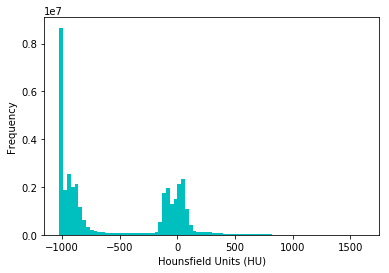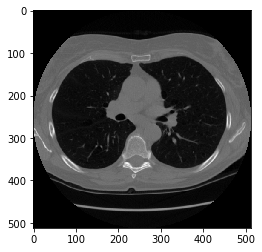Looking at the table from Wikipedia and this histogram, we can clearly see which pixels are air and which are tissue. We will use this for lung segmentation in a bit :)

# Resampling¶

A scan may have a pixel spacing of [2.5, 0.5, 0.5], which means that the distance between slices is 2.5 millimeters. For a different scan this may be [1.5, 0.725, 0.725], this can be problematic for automatic analysis (e.g. using ConvNets)!

A common method of dealing with this is resampling the full dataset to a certain isotropic resolution. If we choose to resample everything to 1mm1mm1mm pixels we can use 3D convnets without worrying about learning zoom/slice thickness invariance.

Whilst this may seem like a very simple step, it has quite some edge cases due to rounding. Also, it takes quite a while.

Below code worked well for us (and deals with the edge cases):

In :
def resample(image, scan, new_spacing=[1,1,1]):
# Determine current pixel spacing
spacing = np.array([scan.SliceThickness] + scan.PixelSpacing, dtype=np.float32)

resize_factor = spacing / new_spacing
new_real_shape = image.shape * resize_factor
new_shape = np.round(new_real_shape)
real_resize_factor = new_shape / image.shape
new_spacing = spacing / real_resize_factor

image = scipy.ndimage.interpolation.zoom(image, real_resize_factor, mode='nearest')

return image, new_spacing


Please note that when you apply this, to save the new spacing! Due to rounding this may be slightly off from the desired spacing (above script picks the best possible spacing with rounding).

Let's resample our patient's pixels to an isomorphic resolution of 1 by 1 by 1 mm.

In :
pix_resampled, spacing = resample(first_patient_pixels, first_patient, [1,1,1])
print("Shape before resampling\t", first_patient_pixels.shape)
print("Shape after resampling\t", pix_resampled.shape)

Shape before resampling	 (134, 512, 512)
Shape after resampling	 (335, 306, 306)


# 3D plotting the scan¶

For visualization it is useful to be able to show a 3D image of the scan. Unfortunately, the packages available in this Kaggle docker image is very limited in this sense, so we will use marching cubes to create an approximate mesh for our 3D object, and plot this with matplotlib. Quite slow and ugly, but the best we can do.

In :
def plot_3d(image, threshold=-300):

# Position the scan upright,
# so the head of the patient would be at the top facing the camera
p = image.transpose(2,1,0)

verts, faces = measure.marching_cubes(p, threshold)

fig = plt.figure(figsize=(10, 10))
ax = fig.add_subplot(111, projection='3d')

# Fancy indexing: verts[faces] to generate a collection of triangles
mesh = Poly3DCollection(verts[faces], alpha=0.70)
face_color = [0.45, 0.45, 0.75]
mesh.set_facecolor(face_color)

ax.set_xlim(0, p.shape)
ax.set_ylim(0, p.shape)
ax.set_zlim(0, p.shape)

plt.show()


Our plot function takes a threshold argument which we can use to plot certain structures, such as all tissue or only the bones. 400 is a good threshold for showing the bones only (see Hounsfield unit table above). Let's do this!

In :
plot_3d(pix_resampled, 400)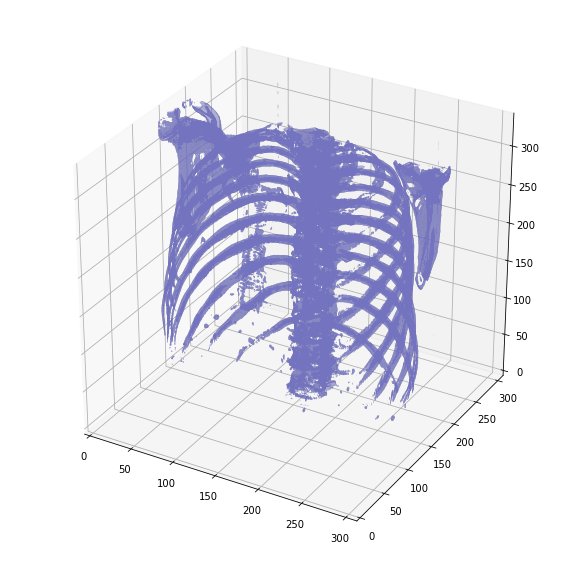Spooky!

# Lung segmentation¶

In order to reduce the problem space, we can segment the lungs (and usually some tissue around it). The method that me and my student colleagues developed was quite effective.

It involves quite a few smart steps. It consists of a series of applications of region growing and morphological operations. In this case, we will use only connected component analysis.

The steps:

• Threshold the image (-320 HU is a good threshold, but it doesn't matter much for this approach)
• Do connected components, determine label of air around person, fill this with 1s in the binary image
• Optionally: For every axial slice in the scan, determine the largest solid connected component (the body+air around the person), and set others to 0. This fills the structures in the lungs in the mask.
• Keep only the largest air pocket (the human body has other pockets of air here and there).
In :
def largest_label_volume(im, bg=-1):
vals, counts = np.unique(im, return_counts=True)

counts = counts[vals != bg]
vals = vals[vals != bg]

if len(counts) > 0:
return vals[np.argmax(counts)]
else:
return None

# not actually binary, but 1 and 2.
# 0 is treated as background, which we do not want
binary_image = np.array(image > -320, dtype=np.int8)+1
labels = measure.label(binary_image)

# Pick the pixel in the very corner to determine which label is air.
#   Improvement: Pick multiple background labels from around the patient
#   More resistant to "trays" on which the patient lays cutting the air
#   around the person in half
background_label = labels[0,0,0]

#Fill the air around the person
binary_image[background_label == labels] = 2

# Method of filling the lung structures (that is superior to something like
# morphological closing)
if fill_lung_structures:
# For every slice we determine the largest solid structure
for i, axial_slice in enumerate(binary_image):
axial_slice = axial_slice - 1
labeling = measure.label(axial_slice)
l_max = largest_label_volume(labeling, bg=0)

if l_max is not None: #This slice contains some lung
binary_image[i][labeling != l_max] = 1

binary_image -= 1 #Make the image actual binary
binary_image = 1-binary_image # Invert it, lungs are now 1

# Remove other air pockets insided body
labels = measure.label(binary_image, background=0)
l_max = largest_label_volume(labels, bg=0)
if l_max is not None: # There are air pockets
binary_image[labels != l_max] = 0

return binary_image

In :
segmented_lungs = segment_lung_mask(pix_resampled, False)
segmented_lungs_fill = segment_lung_mask(pix_resampled, True)

In :
plot_3d(segmented_lungs, 0)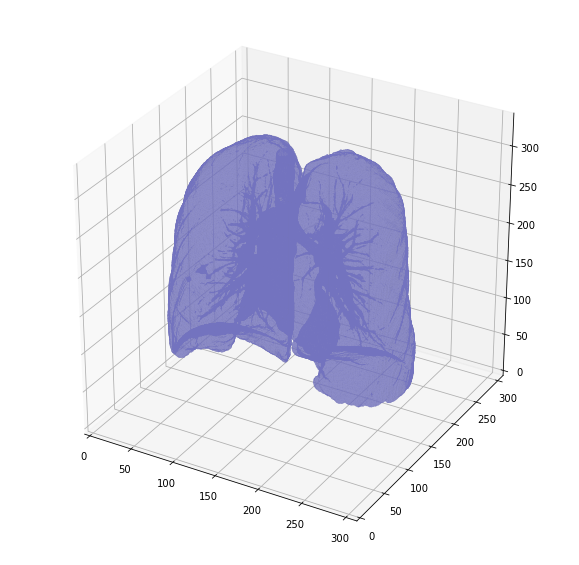Beautiful!

But there's one thing we can fix, it is probably a good idea to include structures within the lung (as the nodules are solid), we do not only want to air in the lungs.

In :
plot_3d(segmented_lungs_fill, 0)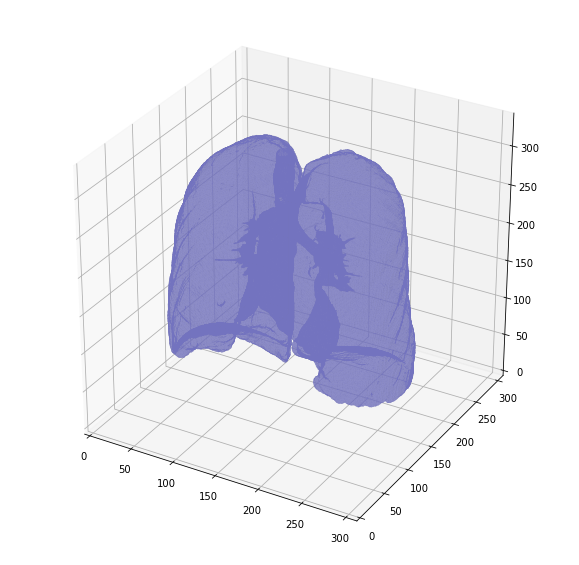That's better. Let's also visualize the difference between the two.

In :
plot_3d(segmented_lungs_fill - segmented_lungs, 0)